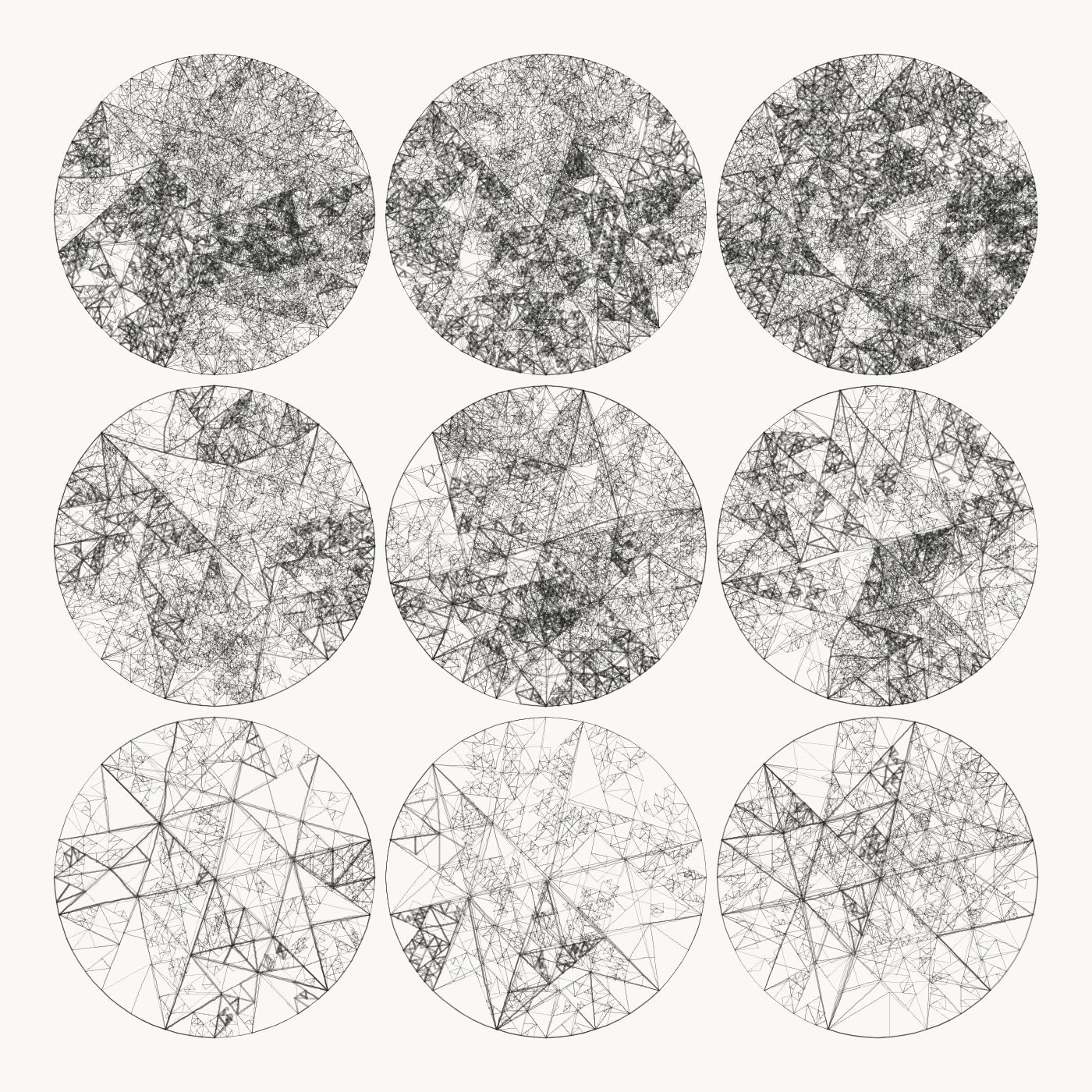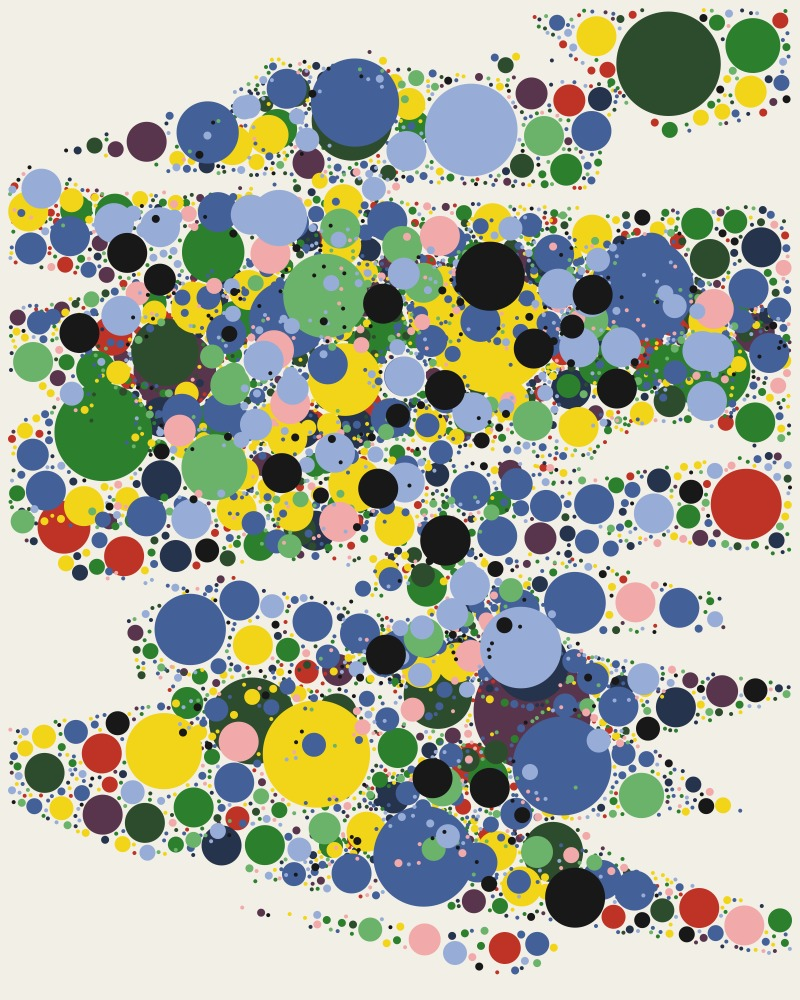# 4. Algorithms & Recursion

## Algorithms for visuals

### Recursion

Basic model:

``````
function doRepeat(){
draw something
doRepeat();
}
``````

However, this recursion would go on forever subsequently crashing the computer. We need a condition to break the infinite execution:

``````
counter = 0;

function doRepeat(){
//draw something here
counter ++;
if (counter < 100){
doRepeat();
}

}
``````

### Recursive devision of areas or volumes

Tyler Hobbs, USAAESTHETICALLY PLEASING TRIANGLE SUBDIVISIONWe can realize a similar approach in P5 using recursions to divide the area of triangles.

### Fractals

Fractals have been defined by Benoit B. Mandelbrot in 1975 in his work “The Fractal Geometry of Nature”. Fractals are a rough or fragmented geometric shape that can be split into parts, each of which is (at least approximately) a reduced-size copy of the whole.

Fractal patterns are often created by recursive operations. Even simple creation rules result in complex patterns after a few recursive steps.

The simplest examples of self-similar objects are lines, parallelograms (including squares) and cubes, because they can be broken down into smaller copies of themselves by cuts parallel to their sides. However, these are not fractals, because their similarity dimension and Lebesgue's overlap dimension are the same. An example of a self-similar fractal is the Sierpinski Triangle

### Koch Curve### Dragon Curve### Sierpinski Triangle

The algorithm that produces a Sierpinski Triangle can be described relatively simple:

• Subdivide it into four smaller congruent equilateral triangles and remove the central triangle.
• Repeat step 2 with each of the remaining smaller triangles infinitely.### L-Systems and TreesImage of computer generated illustrations of weeds.

### Trees

Plant-like structures can easily be simulated be recursive functions. Here is a version in P5:

### Example: Trees, Art-Prints. Andreas Pirchner, 2019### Example: Recursive structures as illustrations in a publication by Austrian Museums Association. Andreas Pirchner, 2019Assignment: Random Sierpinski Triangle
Have a shot on the Sierpinski Triangle! Can you come up with a version of it, that doesn't divide the triangle not in the middle of each its sides but choses a random point?

### Example: Cover-Illustraion, 3D Tree, Processing Octane Render, Andreas Pirchner, 2020

The generative cover illustration for ›neues museum 20-1/2‹. I did the recursive tree-like structure in Processing and added some geometric calculations, that led to additional volumens and objects in the structure. I exported the generative object from Processing and used Octane Render for materials and the rendering.### Circle Packing Algorithm

Another interesting algorithmic way of generating images is circle packing. It can be realized in P5 by including

Pseudo-Code

• Draw a circle somewhere on the canvas.
• See, if it intersects an already existing circle. If yes, move it and check again.
• Grow the circle until it hits another circle.### Leaves and Forces

Assignment: Leaves and Forces
Use what you learned from today`s class and the last session to code a recursive tree using objects for each branch. Can you animate the tree, when you store each branch and the coordinates of the nodes in the objects (and them in an array)?